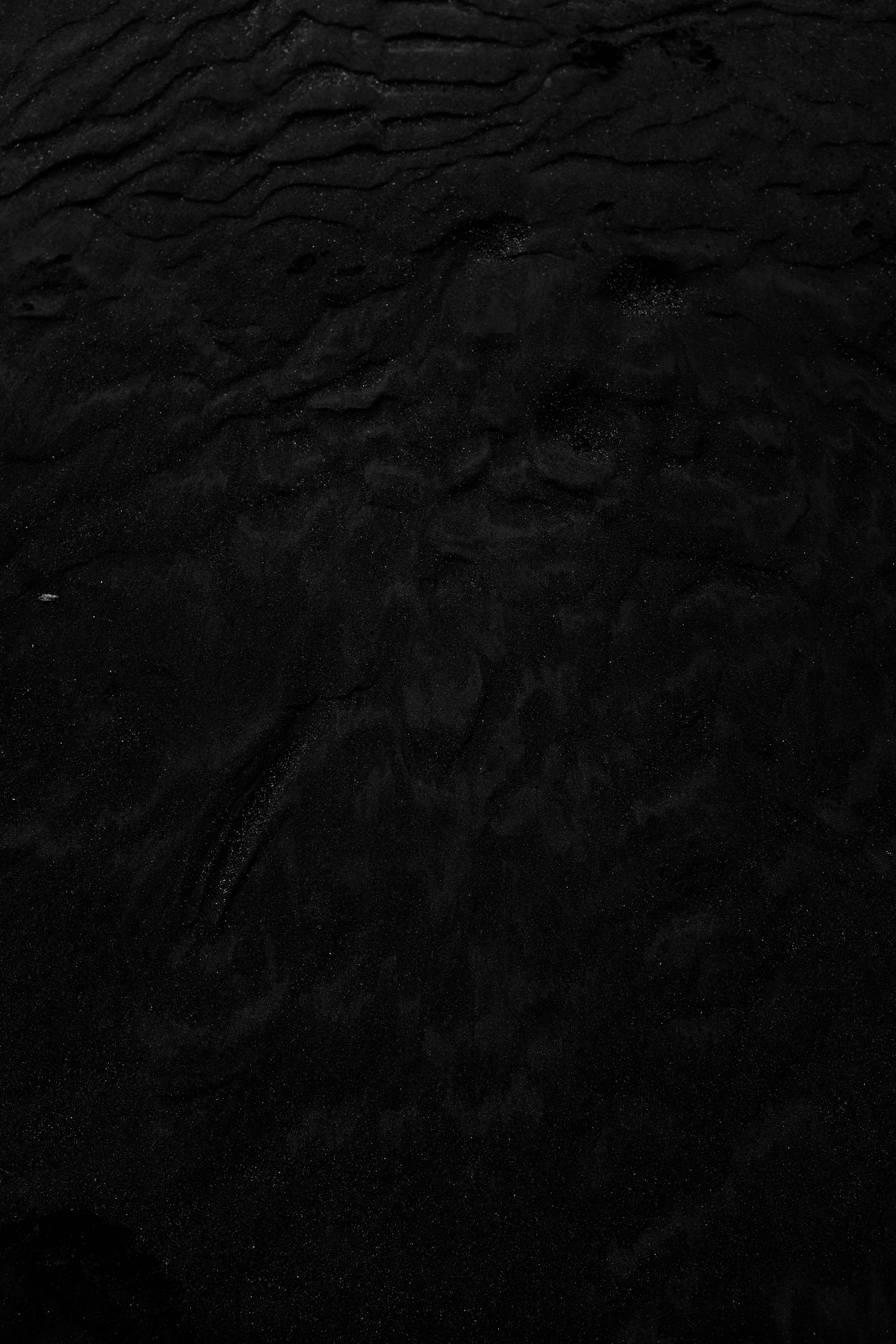~ I . assume ~4
•
0

Autoplay OFF   •   3 months ago
[He.. *zzz* coming*// zz*.. RU..*zz** {REDACTED}

# ~ I . assume ~

I assume the sky is blue

I assume life will [...end..] _co//n'tin-*u*e

I assume time keeps going

I assume we all know

I assume one day _t//h'in-[REDACTED} [He] will change

I assume that I should show

I assume that they would [Find thi...{error]8x666exe}

I assume I should say it - ][will c-come for... y....]

I assume the worse to happen [To you... R...]

I assume I'm different

I assume you can guess.

you can guess..

you can guess... Right? [U//] [/N] [ ! ]

~ I . assume ~

Stories We Think You'll Love 💕